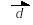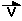#### Kinematics - Describing Motion

There are two types of motion:

• Constant Motion (no acceleration - constant speed)
• Accelerated Motion (motion at changing speed)

Constant Motion obeys two conditions

1. must be in a straight line
2. must be at constant speed

Accelerated Motion

1. does not have to be in a straight line
2. speed changes over time intervals

Distance, Displacement, Speed, Velocity

Displacement is defined as the distance traveled in one direction.

The symbol for displacement is.........  it's a vector quantity.
Displacement is  a vector quantity.

The unit of displacement is the meter (m).  The symbol for distance is d.

Velocity is the speed an object travels in one particular direction.

The symbol for speed  is V and the units for speed are " meters per second" or (m/s).

The symbol for velocity is .......   It's a vector quantity.

To calculate speed (or velocity) we use the equation

The triangle system is a simplified method to rearrange this equation and solve for the other two variables: d, and t

Example:

If you walk your dog at 30 cm/s.  How far will you have traveled in 20 minutes?

Given:  v = 30 cm/s = 0.30 m/s
t = 20 min. = 20 min X 60 s/min = 1200 s

Find: d

..............................  then   d = v X t

Therefore

d = 0.30 m/s  X 1200 s  = 360 m

Therefore you would have walked a distance of 360 m

Acceleration

Acceleration is the rate at which speed increases or decreases.

When an object slows down we say it is decelerating.

Deceleration is negative acceleration.

The symbol for acceleration is a.

The units for acceleration are m/s2

The general simplified equation to calculate the magnitude of the average acceleration of an object is

a = v/t.